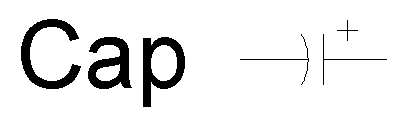Circle

Draw a circle using one of four methods.

Mode Settings:

Setting Data Type Comments
Method Center, Radius | Center, Diameter | Two Points | Three Points Choose method

Center, Radius Method: Construct a circle from a center and radius.

Prompt Data Type Comments
Center Point Center of the circle
Radius Point or Distance > 0 Radius of the circle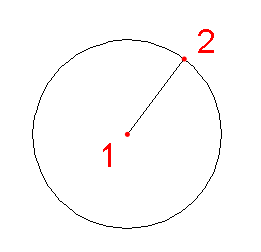Center, Diameter: Construct a circle from a center and diameter.

Prompt Data Type Comments
Center Point Center of the circle
Diameter Point or Distance > 0 Diameter of the circle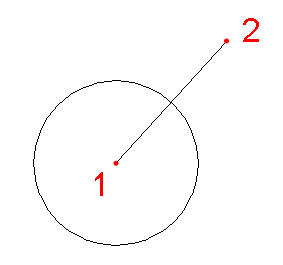Two Points Method: Construct a circle from two points. The center is calculated as the midpoint of the two points.

Prompt Data Type Comments
Point 1 Point
Point 2 Point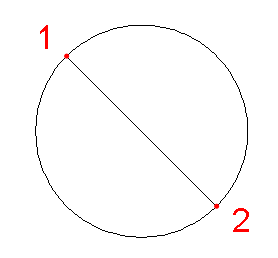Three Points Method: Construct a circle from three points. The circle is guaranteed to pass through all three points.

Prompt Data Type Comments
Point 1 Point
Point 2 Point
Point 3 Point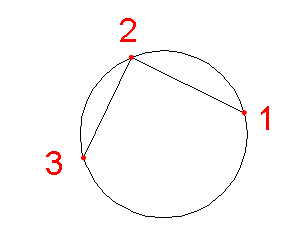Invalid State: Radius <= 0